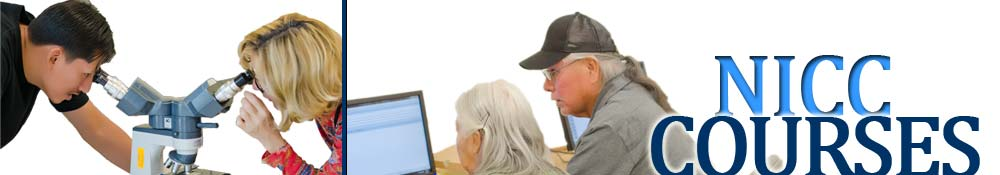## Mathematics Courses offered at NICC

MATH 0900 MATH FOUNDATIONS (3)

Students are offered the opportunity to review and increase mathematics skills in addition, subtraction, multiplication, division, whole numbers, fractions, decimals, percentages, basic algebraic manipulation, and geometry. (Previously known as MTH 099)

MATH 1060 CONSTRUCTION MATH (3)

This course provides applied mathematics for the student enrolled in the carpentry and building construction program. Gives student the ability to use basic math skills to employ in blueprint design, distance measurements, squaring off of floor plans, materials ordering with purchase orders, materials estimates, and the like. (Previously known as MTH 106)

MATH 1110 INTERMEDIATE ALGEBRA (4)

This course will include: polynomials and exponents, factoring, rational expressions, roots and radicals, complex numbers, quadratic equations, systems of linear equations, functions, and logarithms. (Previously known as MTH 111) *Prerequisite: MATH 1100 or equivalent mathematical skill level

MATH 1150 COLLEGE ALGEBRA (4)

This course will include: sets, real and complex numbers, absolute value, exponents and radicals, functions, graphing, solutions of linear, quadratic, rational equations and inequalities, systems of equations, exponential logarithmic and inverse functions, conic sections, sequences and series, and other pre-calculus topics. (Previously known as MTH 112) *Prerequisite: MATH 1110 or equivalent mathematical skill level. Credit will be given for only one: MATH 1150 or 1200

MATH 1200 ALGEBRA AND TRIGONOMETRY (4)

Linear equations and inequalities, relations and functions, graphing of polynomials and rational functions, logarithms and exponential functions, systems of equations, trigonometric functions and their applications. (Previously known as MTH 120) *Prerequisite: Two years high school mathematics or “C” grade or better in MATH 1100

MATH 2020 GEOMETRY FOR ELEMENTARY SCHOOL TEACHERS (3)

Fundamental mathematical concepts basic to the understanding of elementary geometry will be presented in this course. (Previously known as MTH 202) *Prerequisite: Teacher or child development program students or approval by instructor.

MATH 2030 CONTEMPORARY MATHEMATICS (3)

Applications of quantitative reasoning and methods to problems and decision making in the areas of management, statistics, and social choice. Topics include networks, critical paths, linear programming, sampling, central tendency, inference, voting methods, power index, game theory, and fair decision problems. (Previously known as MTH 203) *Prerequisite: Teacher program students or approval by instructor

MATH 2170 STATISTICS (4)

The course is an introduction to basic probability and statistical methods that are used in a wide variety of disciplines. Topics include descriptive statistics, probability foundations, probability distributions, sampling distributions, methods of statistical inference, and bivariate relationships. (Previously known as MTH 215) *Prerequisite: MATH 1110- Intermediate Algebra or appropriate math placement

MATH 2300 MATH FOR ELEMENTARY TEACHERS (3)

This course will cover numeration, sets, relations, bases, and a detailed development of the number system from an elementary school point of view. A key component of this course will be to learn about and create good habits relating to the five NCTM process standards: problem solving, communication, representation, reasoning and proof, and connections. (Previously known as MTH 230) *Prerequisite: MATH 1110

MATH 2400 BUSINESS CALCULUS (3)

This course is an accelerated course in applications of mathematics to business and economics. Topic coverage include: 1) equations and their graphs; 2) matrices and their operations; 3) differential calculus, in particular, first and second derivatives of single and multi-variable functions; and 4) the modeling of business and economic problems. Computer applications are included. (Previously known as MTH 240) *Prerequisite: MATH 1110

MATH 2500 CALCULUS I (5)

Plane analytical geometry, theory of limits, derivatives and applications, integrals.
(Previously known as MTH 250) *Prerequisite: MATH 1150

MATH 2900 SPECIAL TOPICS (1-3)

(Previously known as MTH 290)

MATH 2990 INTERNSHIP (1-4)

Internship

### Academic Information programs, courses & transfer info.